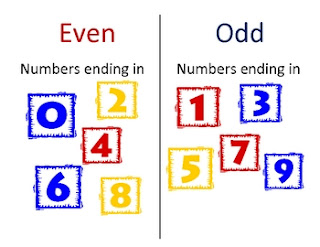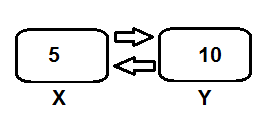Print all odd number using c programmingOdd and Even number in c
Before doing the code to find all odd number using c programming, we have to know the logic behind the code. We have discussed the logical part. Now it's time to write some code. But I suggest, please go back to the logical part of the code first. The logical thinking to find odd and even number is the same.

Print all even number using c programming

Before doing code to find all even number using c programming, we have to know the logic behind the code. We have discuss about the logical part. Now it's time to write some code. But I suggest, please go back to the logical part of the code first.Even and Odd number

Print all even number in c programming

To print all even number from a range in c programming, we have to check all number between given range. To check if the number is odd or even number, we can use this following logic:
The rule is, if any number is fully divided by 2, then this number is even. Else the number is odd.Even and Odd

For example

If we want to check whether the number 5 odd or even, we need to simply divide the number by 2.
If there is any reminder left, or if there is any value of 5 mod 2 left, that means 5 is not divisible by 2. So 5 is odd number.

How to swap 2 number in c programming?

We have discuss about the logic behind this code to solve our problem. If you missed the basic logical part, please go back and understand the logic and then try to code yourself.Swap two number

How to swap 2 number ?

There is two number in two variable. We have to swap the position of the numbers. For example, theSwap
variable x holding integer value 5 and another variable y holding the integer value 10. Now we have to swap the position of those two numbers.

Modulus in c programmingModulus
Finding modulus in c programming is so easy. We just need to use % to find mod.
For example, if the question is X mod Y, we need to simply use X % Y.
The following code calculate the modulus value:

Find modulus:

At first, the question is what is modulus?Modulus
Modulus is the remainder after division of one number by another number. Modulus is also called remainder. For example if we try to divide 5 by 2, we will have some remainder or modulus.
5/2 = 2 and 1 is remainder. Modulus is define by mod.
We can find this remainder easily in c programming. We also can find quotient easily both calculator and using c programming. But sometimes there is no mod button in our scientific calculator. So question is, how to find modulus by simple calculation.

Find the largest number:

The logic of finding a large number is so easy.
We have to declare some variables and just compare them with each other.For example, we have 3 number to compare and we have to find the biggest number from them. The logic of comparing 3 number and find the biggest one is pretty simple.
Think, we have number 5, 15 and 10. So we can first compare the first two number with a condition like, is (5 > 15)? If this condition is true, then we will take 5 as the bigger number. But here the condition is not true.
5 is not bigger than 15. So there will be other condition like:

PHP MySql tutorial: part 1

After go through PHP MySql, let's create a form to make our database look better. We will try to create a database using PHP MySql. In which, we can insert some data. It can be student information system.The form will looks like bellow:

Let's create the form which is not really useful for the first time.
I have consider that you know the basic tags of HTML.
Let's do some HTML code then I will explain:

let me explain something. Here we have use some tag in our code.
We have use:
# 1 : <form> ---- </form>  to create a form
# 2 : name = "form" to define name, it could be anything, but it must be similar to the name of the database. For example, if the name of the database is "studentInfo", then the name="studentInfo" is alright.
# 3 : action = "index.php" action is the next task to do.
# 4 : method="POST" mean that, the form data will be posted into the database.
There is two method. One to GET method and another is POST. Get is use to get something and post is use to posting something in a common sense.
# 5 : Then <fieldset> --- </fieldset> use to create a separate field. The <input> --- </input> use to perform the action. We use type as submit which will create a submit button and pass the value to the database.
I hope this article is understandable to learn the first step of PHP MySql. Follow the full tutorial series to learn PHP MySql database.

HTML Bangla pdf e-BookCSS Bangla e-book

Next tutorial ( what is XAMPP and Install XAMPP in computer) coming soon...
If you have any question or suggestion this post( PHP MySql: Creating a form in HTML) , please comment bellow.

Quick sort algorithm implementation in java

As the name, quick sort is the most quick algorithm to sort some number. There are some other sorting algorithm like bucket sort, insertion sort, bubble sort, radix sort etc. Among them, quick sort is very efficient.
Here is the step diagram of quick sort:
Let's look at the code:

Data Communications and NetworkingBy Behrouz A.Forouzan free pdf book

This is one of the best book for data communication and networking.